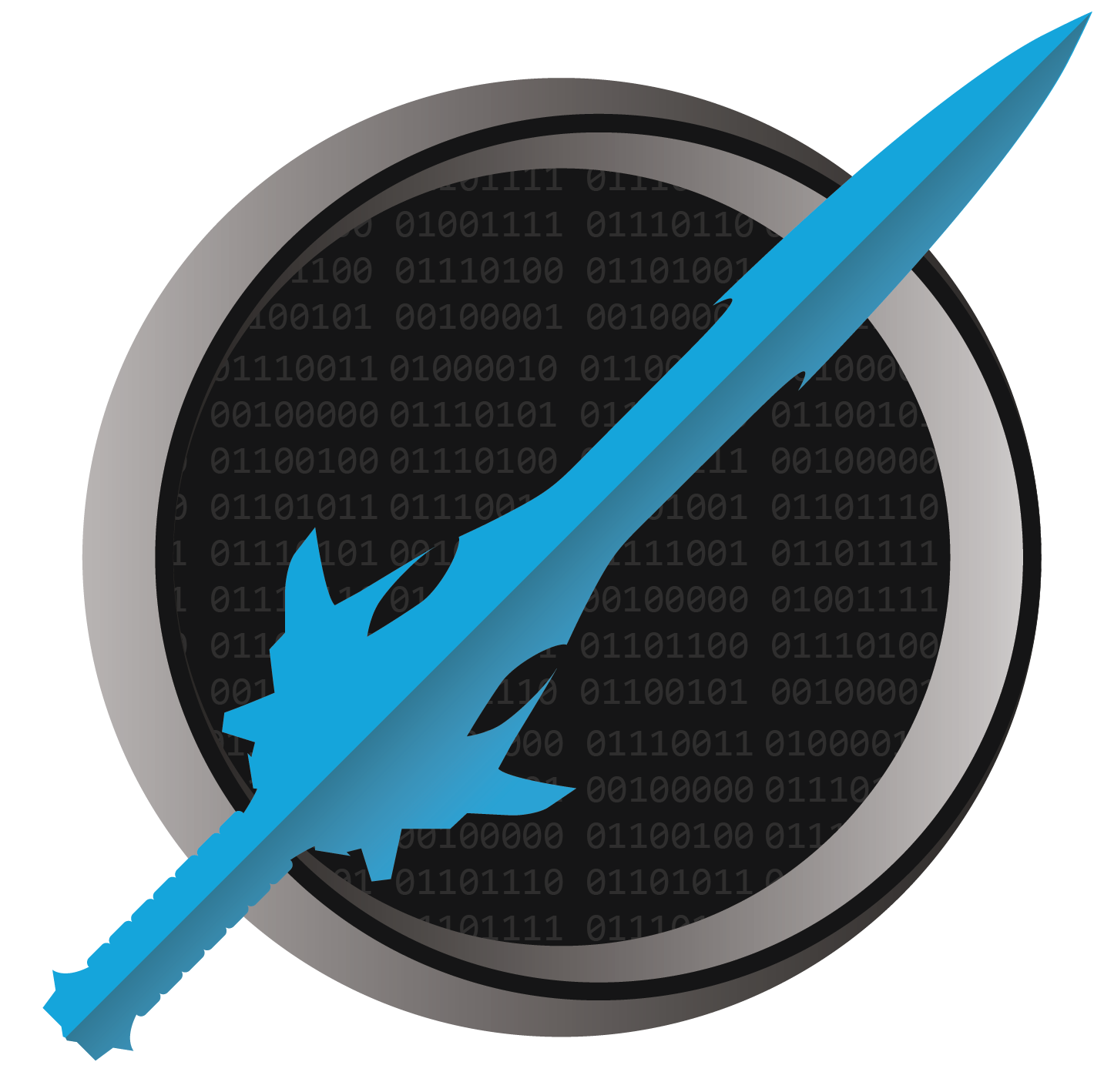It's dangerous to code alone! Take this.

Knowledge Check - Type System

1. True/False. The int type can store any possible integer.

False. It has a range (roughly -2 billion to +2 billion). It cannot store numbers beyond that range.

2. Order the following by how large of numbers they can hold, from smallest to largest: short, long, int, byte.

byte, short, int, long. A byte is 1 byte and its largest value is 255. A short is 2 bytes and its largest value is approximately 32 thousand. An int is 4 bytes and its largest value is approximately +2 billion. A long is 8 bytes, and its largest value is approximately 9 quintillion.

3. True/False. The byte type is signed.

False. A signed type is one that includes a positive or negative sign. A byte is the range 0 to 255, and does not allow for negative values. Thus, it is unsigned.

4. Which can store higher numbers, int or uint?

uint. Both are 4 bytes in size, but int’s range is about -2 billion to +2 billion, while uint is 0 to about 4 billion. That makes uint capable of storing larger numbers, but int capable of storing negative numbers that uint cannot store.

5. What three types can store floating-point numbers?

The three floating-point types are float, double, and decimal. These store numbers that can include a decimal point.

6. Which of the options in question 5 can hold the largest numbers?

double holds the biggest numbers, though decimal uses more bytes and can represent more precise numbers, even though its range isn’t as large.

7. Which of the options in question 5 is considered the most precise?

decimal is the most precise of the three, though double can store larger numbers.

8. What type does the literal value "8" (including the quotes) have?

A literal "8" has a type of string. Even though it looks like a digit, it is textual because it is in quotes. And because it is double-quotes instead of single quotes, it is a string literal.

9. What type stores true or false values?

The bool type is for storing values of true and false.